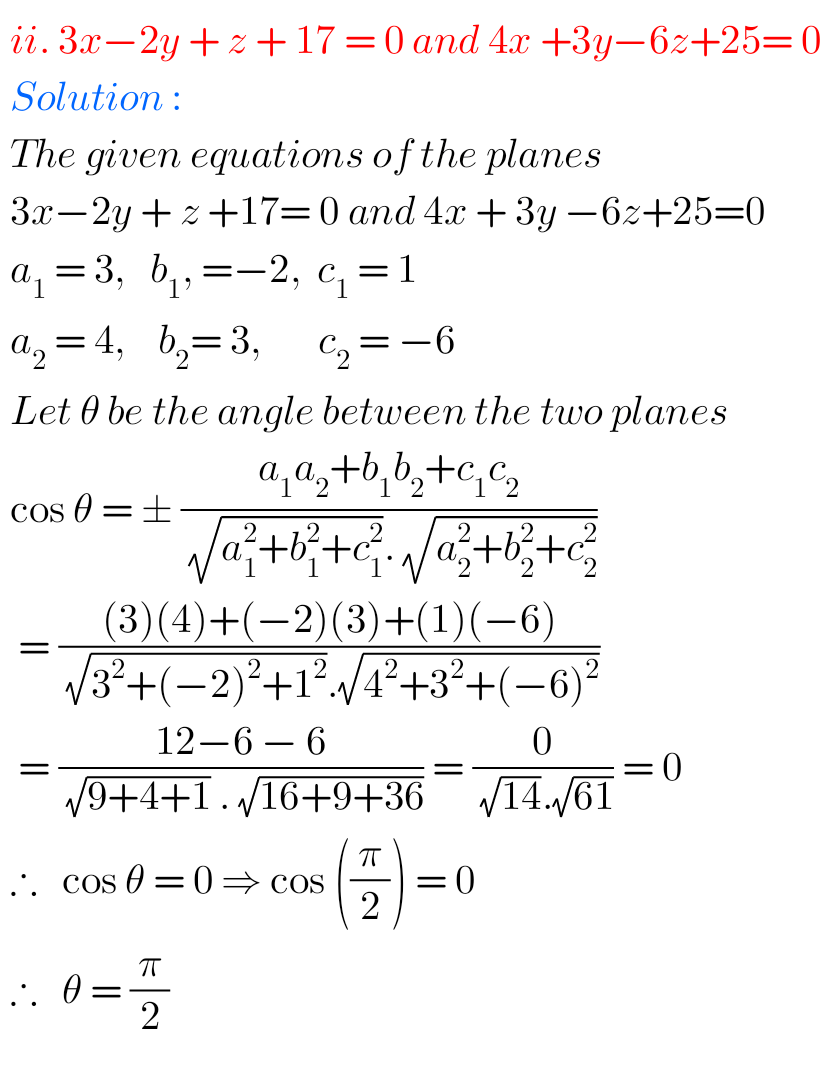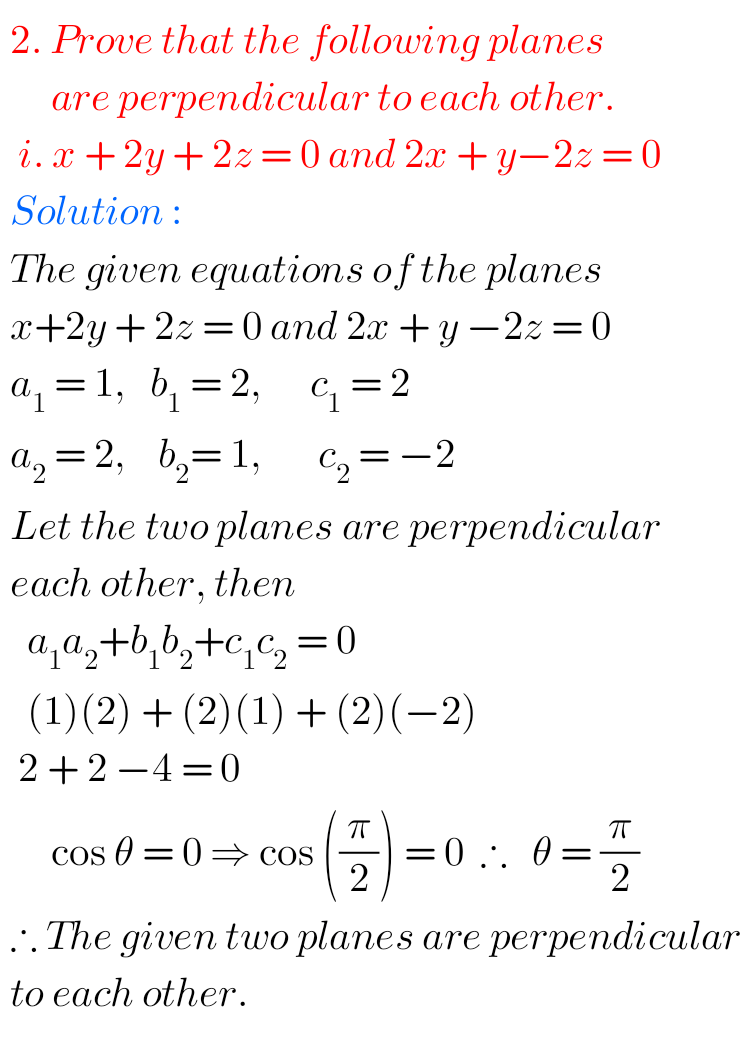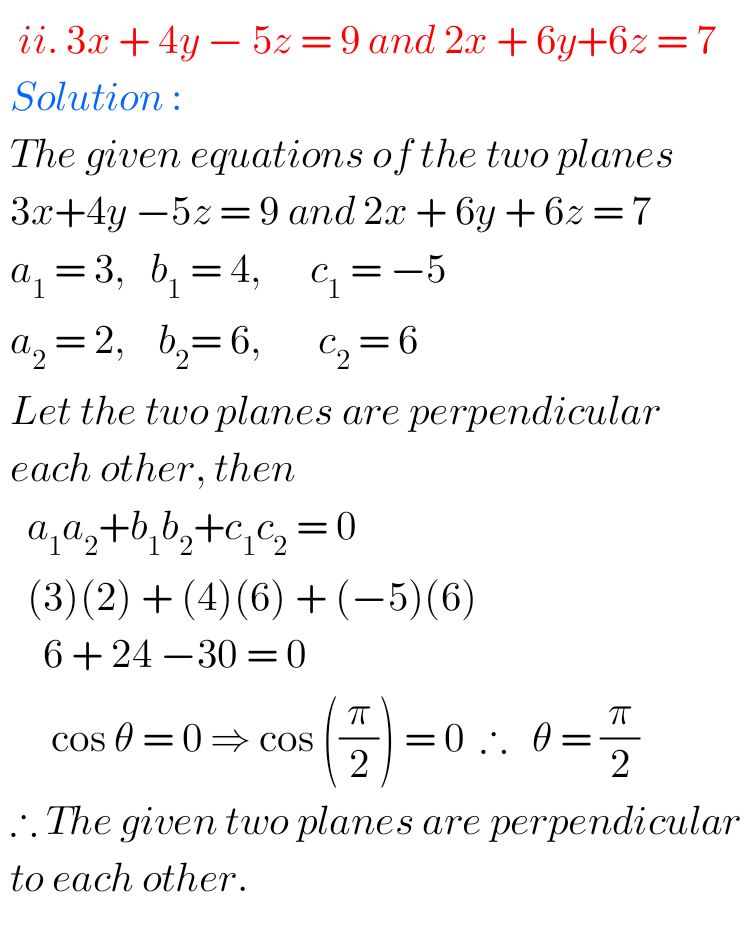# Plane check your progress 35.2 solutions NIOS class 12

NIOS Mathematics 311 book 2 Module IX Chapter 36 Plane check your progress 35.2 solutions are given.

You should study the textbook lesson Plane very well.

You should also observe and practice all problems and solutions given in the textbook.

Observe the given solutions and try them in your own method.

You can also see.

NIOS Maths 311 book 2 solutions for some chapters

## Maths 311 book 2 chapter 35 Plane check your progress 35.2 solutions NIOS

Plane

Chapter 35

1. Find the angle between the planes: i. 2x -y + z = 6 and x + y + 2z = 3ii. 3x -2y + z + 17 = 0 and 4x + 3y -6 + 25 = 02. Prove that the following are perpendicular to each other: i. x + 2y + 2z = 0 and 2x + y -2z = 0.ii. 3x + 4y -5 = 9 and 2x + 6y + 6x = 7m

### NIOS maths 311 Plane solutions check your progress 35.2

m

Note: Observe the solutions and try them in your own method.

Inter maths solutions

SSC maths class 10 solutions

Ncert maths class 6 solutions